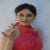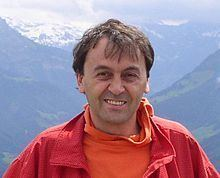# Giuseppe Melfi

Updated on
Covid-19
 Nationality  Italy   SwitzerlandFields  MathematicsRole  Mathematician Name  Giuseppe MelfiBorn  11 June 1967 (age 48) Uznach, Switzerland (1967-06-11) Institutions  University of Neuchatel University of Applied Sciences Western Switzerland University of Teacher Education BEJUNEKnown for  Practical numbers Ramanujan-type identitiesInstitution  University of Neuchatel, University of Applied Sciences Western Switzerland Notable awards  Premio Ulisse (2010)

Giuseppe Melfi (born June 11, 1967) is an Italo-Swiss mathematician. He achieved his PhD in mathematics in 1997 at the University of Pisa. After some years spent at the University of Lausanne, he works now at the University of Neuchâtel, as well as at the University of Applied Sciences Western Switzerland and at the local University of Teacher Education.

## His work

His major contributions are in the field of practical numbers. This prime-like sequence of numbers is known for having an asymptotic behavior and other distribution properties similar to the sequence of primes. Melfi proved two conjectures both raised in 1984 one of which is the corresponding of the Goldbach conjecture for practical numbers: every even number is a sum of two practical numbers. He also proved that there exist infinitely many triples of practical numbers of the form m 2 , m , m + 2 .

Another notable contribution has been in an application of the theory of modular forms, where he found new Ramanujan-type identities for the sum-of-divisor functions. In particular he found the remarkable identity

k = 0 n σ 1 ( 3 k + 1 ) σ 1 ( 3 n 3 k + 1 ) = 1 9 σ 3 ( 3 n + 2 ) ,

where σ k ( n ) is the sum of the k -th powers of divisors of n .

Among other problems in elementary number theory, he is the author of a theorem that allowed him to get a 5328-digit number that is at present the largest known primitive weird number.

In applied mathematics his research interests include probability and simulation.

## Selected research publications

• Giuseppe Melfi and Yadolah Dodge (2008). Premiers pas en simulation. Springer-Verlag. ISBN 978-2-287-79493-3.
• Deshouillers, Jean-Marc; Erdős, Pál; Melfi, Giuseppe (1999), "On a question about sum-free sequences", Discrete Mathematics, 200: 49–54, MR 1692278, doi:10.1016/s0012-365x(98)00322-7 .
• Melfi, Giuseppe (2015). "On the conditional infiniteness of primitive weird numbers". Journal of Number Theory. Elsevier. 147: 508–514. doi:10.1016/j.jnt.2014.07.024.
• Melfi, Giuseppe (1996), "On two conjectures about practical numbers", Journal of Number Theory, 56 (1): 205–210, MR 1370203, doi:10.1006/jnth.1996.0012
• Giuseppe Melfi Wikipedia

Topics